Particle Physics and Cosmology

# 86 The Big Bang

### Learning Objectives

By the end of this section, you will be able to:

• Explain the expansion of the universe in terms of a Hubble graph and cosmological redshift
• Describe the analogy between cosmological expansion and an expanding balloon
• Use Hubble’s law to make predictions about the measured speed of distant galaxies

We have been discussing elementary particles, which are some of the smallest things we can study. Now we are going to examine what we know about the universe, which is the biggest thing we can study. The link between these two topics is high energy: The study of particle interactions requires very high energies, and the highest energies we know about existed during the early evolution of the universe. Some physicists think that the unified force theories we described in the preceding section may actually have governed the behavior of the universe in its earliest moments.

### Hubble’s Law

In 1929, Edwin Hubble published one of the most important discoveries in modern astronomy. Hubble discovered that (1) galaxies appear to move away from Earth and (2) the velocity of recession (v) is proportional to the distance (d) of the galaxy from Earth. Both v and d can be determined using stellar light spectra. A best fit to the sample illustrative data is given in (Figure). (Hubble’s original plot had a considerable scatter but a general trend was still evident.)

This graph of red shift versus distance for galaxies shows a linear relationship, with larger red shifts at greater distances, implying an expanding universe. The slope gives an approximate value for the expansion rate. (credit: John Cub)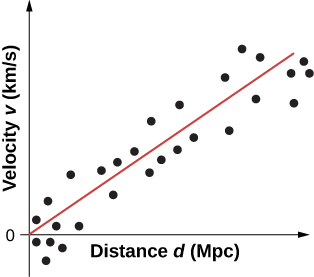The trend in the data suggests the simple proportional relationship: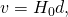where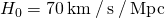is known as Hubble’s constant. (Note: 1 Mpc is one megaparsec or one million parsecs, where one parsec is 3.26 light-years.) This relationship, called Hubble’s law, states that distant stars and galaxies recede away from us at a speed of 70 km/s for every one megaparsec of distance from us. Hubble’s constant corresponds to the slope of the line in (Figure). Hubble’s constant is a bit of a misnomer, because it varies with time. The value given here is only its value today.

Watch this video to learn more about the history of Hubble’s constant.

Hubble’s law describes an average behavior of all but the closest galaxies. For example, a galaxy 100 Mpc away (as determined by its size and brightness) typically moves away from us at a speed of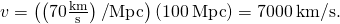This speed may vary due to interactions with neighboring galaxies. Conversely, if a galaxy is found to be moving away from us at speed of 100,000 km/s based on its red shift, it is at a distance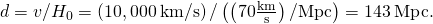This last calculation is approximate because it assumes the expansion rate was the same 5 billion years ago as it is now.

### Big Bang Model

Scientists who study the origin, evolution, and ultimate fate of the universe (cosmology) believe that the universe began in an explosion, called the Big Bang, approximately 13.7 billion years ago. This explosion was not an explosion of particles through space, like fireworks, but a rapid expansion of space itself. The distances and velocities of the outward-going stars and galaxies permit us to estimate when all matter in the universe was once together—at the beginning of time.

Scientists often explain the Big Bang expansion using an inflated-balloon model ((Figure)). Dots marked on the surface of the balloon represent galaxies, and the balloon skin represents four-dimensional space-time (Relativity). As the balloon is inflated, every dot “sees” the other dots moving away. This model yields two insights. First, the expansion is observed by all observers in the universe, no matter where they are located. The “center of expansion” does not exist, so Earth does not reside at the “privileged” center of the expansion (see (Figure)).

An analogy to the expanding universe: The dots move away from each other as the balloon expands; compare (a) to (b) after expansion.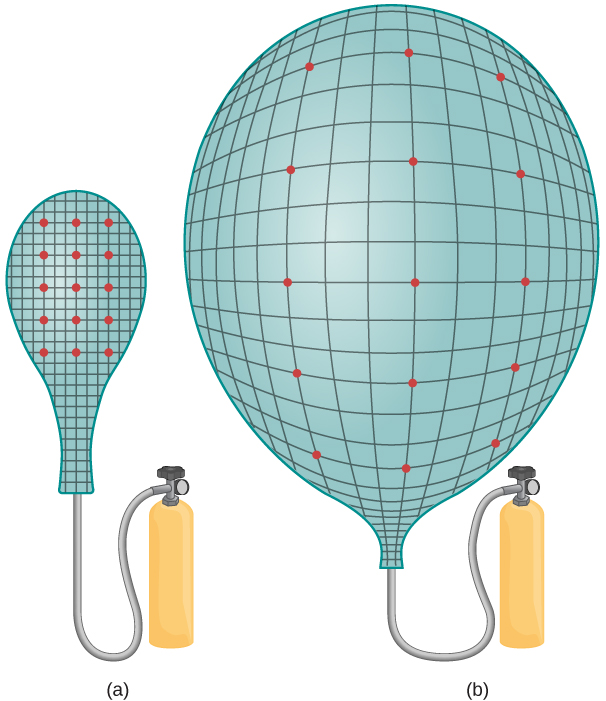Second, as mentioned already, the Big Bang expansion is due to the expansion of space, not the increased separation of galaxies in ordinary (static) three-dimensional space. This cosmological expansion affects all things: dust, stars, planets, and even light. Thus, the wavelength of light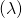emitted by distant galaxies is “stretched” out. This makes the light appear “redder” (lower energy) to the observer—a phenomenon called cosmological redshift. Cosmological redshift is measurable only for galaxies farther away than 50 million light-years.

Calculating Speeds and Galactic Distances A galaxy is observed to have a redshift: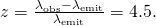This value indicates a galaxy moving close to the speed of light. Using the relativistic redshift formula (given in Relativity), determine (a) How fast is the galaxy receding with respect to Earth? (b) How far away is the galaxy?

Strategy We need to use the relativistic Doppler formula to determine speed from redshift and then use Hubble’s law to find the distance from the speed.

Solution

1. According to the relativistic redshift formula: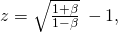where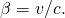Substituting the value for z and solving for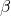, we get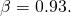This value implies that the speed of the galaxy is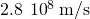.

2. Using Hubble’s law, we can find the distance to the galaxy if we know its
recession velocity: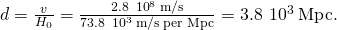Significance Distant galaxies appear to move very rapidly away from Earth. The redshift of starlight from these galaxies can be used to determine the precise speed of recession, over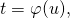of the speed of light in this case. This motion is not due to the motion of galaxy through space but by the expansion of space itself.

Check Your Understanding The light of a galaxy that moves away from us is “redshifted.” What occurs to the light of a galaxy that moves toward us?

blueshifted

View this video to learn more about the cosmological expansion.

### Structure and Dynamics of the Universe

At large scales, the universe is believed to be both isotropic and homogeneous. The universe is believed to isotropic because it appears to be the same in all directions, and homogeneous because it appears to be the same in all places. A universe that is isotropic and homogeneous is said to be smooth. The assumption of a smooth universe is supported by the Automated Plate Measurement Galaxy Survey conducted in the 1980s and 1900s ((Figure)). However, even before these data were collected, the assumption of a smooth universe was used by theorists to simplify models of the expansion of the universe. This assumption of a smooth universe is sometimes called the cosmological principle.

The Automated Plate Measurement (APM) Galaxy Survey. Over 2 million galaxies are depicted in a region 100 degrees across centered toward the Milky Way’s south pole. (credit: 2MASS/T. H. Jarrett, J. Carpenter, & R. Hurt)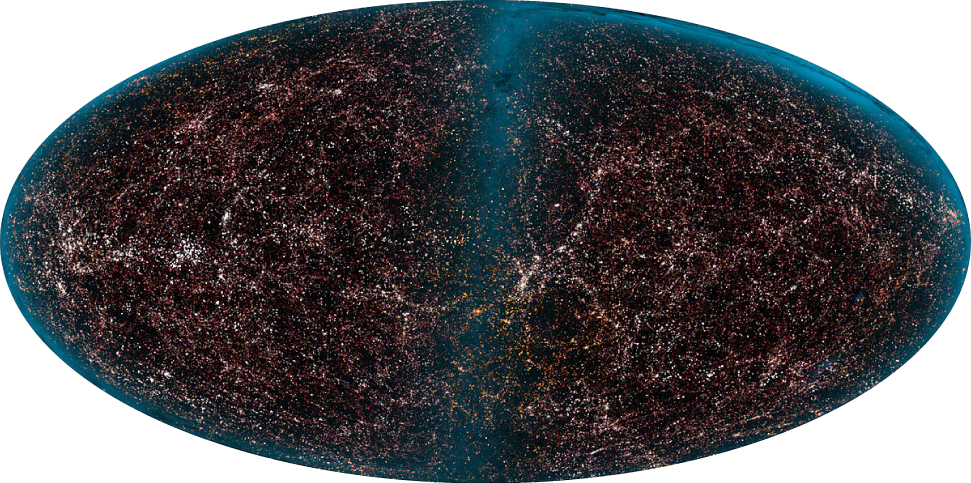The fate of this expanding and smooth universe is an open question. According to the general theory of relativity, an important way to characterize the state of the universe is through the space-time metric: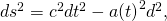where c is the speed of light, a is a scale factor (a function of time), and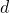is the length element of the space. In spherical coordinates (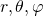, this length element can be written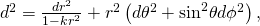where k is a constant with units of inverse area that describes the curvature of space. This constant distinguishes between open, closed, and flat universes:

•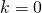(flat universe)
•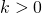(closed universe, such as a sphere)
•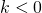(open universe, such as a hyperbola)

In terms of the scale factor a, this metric also distinguishes between static, expanding, and shrinking universes:

•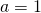(static universe)
•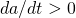(expanding universe)
•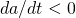(shrinking universe)

The scale factor a and the curvature k are determined from Einstein’s general theory of relativity. If we treat the universe as a gas of galaxies of density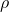and pressure p, and assume(a flat universe), than the scale factor a is given by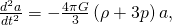where G is the universal gravitational constant. (For ordinary matter, we expect the quantity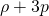to be greater than zero.) If the scale factor is positive (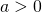), the value of the scale factor “decelerates” (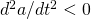), and the expansion of the universe slows down over time. If the numerator is less than zero (somehow, the pressure of the universe is negative), the value of the scale factor “accelerates,” and the expansion of the universe speeds up over time. According to recent cosmological data, the universe appears to be expanding. Many scientists explain the current state of the universe in terms of a very rapid expansion in the early universe. This expansion is called inflation.

### Summary

• The universe is expanding like a balloon—every point is receding from every other point.
• Distant galaxies move away from us at a velocity proportional to its distance. This rate is measured to be approximately 70 km/s/Mpc. Thus, the farther galaxies are from us, the greater their speeds. These “recessional velocities” can be measure using the Doppler shift of light.
• According to current cosmological models, the universe began with the Big Bang approximately 13.7 billion years ago.

### Conceptual Questions

What is meant by cosmological expansion? Express your answer in terms of a Hubble graph and the red shift of distant starlight.

Cosmological expansion is an expansion of space. This expansion is different than the explosion of a bomb where particles pass rapidly through space. A plot of the recessional speed of a galaxy is proportional to its distance. This speed is measured using the red shift of distant starlight.

Describe the balloon analogy for cosmological expansion. Explain why it only appears that we are at the center of expansion of the universe.

Distances to local galaxies are determined by measuring the brightness of stars, called Cepheid variables, that can be observed individually and that have absolute brightnesses at a standard distance that are well known. Explain how the measured brightness would vary with distance, as compared with the absolute brightness.

With distance, the absolute brightness is the same, but the apparent brightness is inversely proportional to the square of its distance (or by Hubble’s law recessional velocity).

### Problems

If the speed of a distant galaxy is 0.99c, what is the distance of the galaxy from an Earth-bound observer?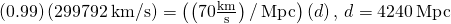The distance of a galaxy from our solar system is 10 Mpc. (a) What is the recessional velocity of the galaxy? (b) By what fraction is the starlight from this galaxy redshifted (that is, what is its z value)?

If a galaxy is 153 Mpc away from us, how fast do we expect it to be moving and in what direction?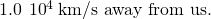On average, how far away are galaxies that are moving away from us at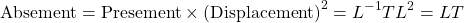of the speed of light?

Our solar system orbits the center of the Milky Way Galaxy. Assuming a circular orbit 30,000 ly in radius and an orbital speed of 250 km/s, how many years does it take for one revolution? Note that this is approximate, assuming constant speed and circular orbit, but it is representative of the time for our system and local stars to make one revolution around the galaxy.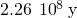(a) What is the approximate velocity relative to us of a galaxy near the edge of the known universe, some 10 Gly away? (b) What fraction of the speed of light is this? Note that we have observed galaxies moving away from us at greater than 0.9c.

(a) Calculate the approximate age of the universe from the average value of the Hubble constant,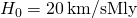. To do this, calculate the time it would take to travel 0.307 Mpc at a constant expansion rate of 20 km/s. (b) If somehow acceleration occurs, would the actual age of the universe be greater or less than that found here? Explain.

a.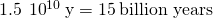; b. Greater, since if it was moving slower in the past it would take less more to travel the distance.

The Andromeda Galaxy is the closest large galaxy and is visible to the naked eye. Estimate its brightness relative to the Sun, assuming it has luminosity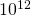times that of the Sun and lies 0.613 Mpc away.

Show that the velocity of a star orbiting its galaxy in a circular orbit is inversely proportional to the square root of its orbital radius, assuming the mass of the stars inside its orbit acts like a single mass at the center of the galaxy. You may use an equation from a previous chapter to support your conclusion, but you must justify its use and define all terms used.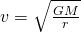### Glossary

Big Bang
rapid expansion of space that marked the beginning of the universe
cosmology
study of the origin, evolution, and ultimate fate of the universe
Hubble’s constant
constant that relates speed and distance in Hubble’s law
Hubble’s law
relationship between the speed and distance of stars and galaxies
redshift
lengthening of the wavelength of light (or reddening) due to cosmological expansion

## LicenseUniversity Physics Volume 3 by cnxuniphysics is licensed under a Creative Commons Attribution 4.0 International License, except where otherwise noted.